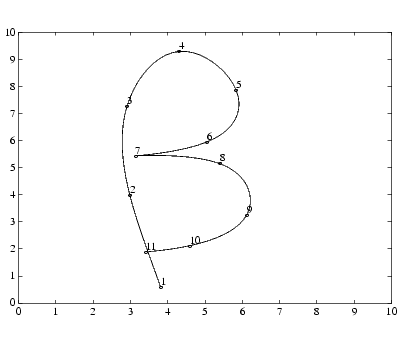GraphicsInteractive Plotting

The `ginput` function enables you to use the mouse or the arrow keys to select points to plot. `ginput` returns the coordinates of the pointer's position, either the current position or the position when a mouse button or key is pressed. See the `ginput` function for more information.

Example -- Selecting Plotting Points from the Screen

This example illustrates the use of `ginput` with the `spline` function to create a curve by interpolating in two dimensions.

First, select a sequence of points, `[x,y]`, in the plane with `ginput`. Then pass two one-dimensional splines through the points, evaluating them with a spacing one-tenth of the original spacing.

• ``````axis([0 10 0 10])
``````hold on
``````% Initially, the list of points is empty.
``````xy = [];
``````n = 0;
``````% Loop, picking up the points.
``````disp('Left mouse button picks points.')
``````disp('Right mouse button picks last point.')
``````but = 1;
``````while but == 1
``````    [xi,yi,but] = ginput(1);
``````    plot(xi,yi,'ro')
``````    n = n+1;
``````    xy(:,n) = [xi;yi];
``````end
``````% Interpolate with a spline curve and finer spacing.
``````t = 1:n;
``````ts = 1: 0.1: n;
``````xys = spline(t,xy,ts);
```
```% Plot the interpolated curve.
``````plot(xys(1,:),xys(2,:),'b-');
``````hold off
``````

This plot shows some typical output.Displaying Contours in Polar Coordinates Animation© 1994-2005 The MathWorks, Inc.